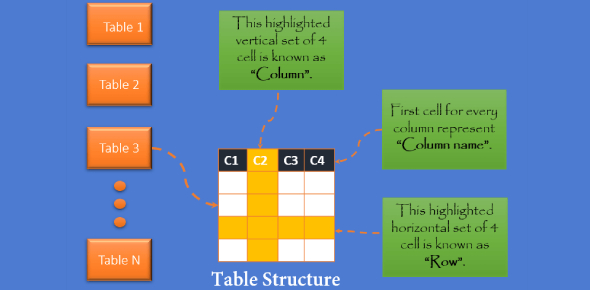# MS Access: Data Processing Quiz!

25 Questions | Attempts: 771
ShareSettings.

• 1.
Which of the following is NOT a part of a database?
• A.

Tables

• B.

Records

• C.

Fields

• D.

Worksheets

• 2.
In a database, each _________________ contains a collection of related data.
• A.

Table

• B.

Record

• C.

Field

• D.

None of the above

• 3.
In a database, a _____________ is a unit of information about one individual or item.
• A.

Table

• B.

Record

• C.

Field

• D.

File

• 4.
Which of the following is a way to sort data?
• A.

Chronologically

• B.

Alphabetically

• C.

Numerically

• D.

All of the above

• E.

None of the above

• 5.
What is a query?
• A.

A question you ask a database

• B.

A question you ask a record

• C.

A question you ask a table

• D.

A question you ask a field

• 6.
A database is an organized collection of information.
• A.

True

• B.

False

• 7.
...............describes the structures of the data within a given domain.
• A.

Data structure

• B.

Data organization

• C.

Data model

• D.

Data management

• 8.
The techniques and tools for capturing and translating complex system designs into easily understood representation are called?
• A.

Data model

• B.

Data modeling

• C.

Data processing

• D.

Option A &C

• 9.
A data model depicts the dataflow and logical interrelationship among different data elements.
• A.

True

• B.

False

• 10.
IDEAS group is the standard for………
• A.

Computer architecture

• B.

Data integration

• C.

Military enterprise

• D.

All the options are correct

• 11.
The ISO standard for computer interpretation, representation, and exchange of product manufacturing information is…….
• A.

ISO10303

• B.

ISO15926

• C.

ISO30310

• D.

ISO92615

• 12.
…….allows you to select and filter data from multiple tables.
• A.

Access file

• B.

Forms

• C.

Queries

• D.

Search engine

• 13.
Which of these is not a sign of data models?
• A.

Help in structuring and organising data

• B.

Facilitate user-end communication

• C.

Foster and improve understanding of organization

• D.

Impose only implicitly constraints on data within structure

• 14.
An example of the tabular details of all your class member’s age, sex, state of original e.t.c is……….
• A.

Record

• B.

Table

• C.

Form

• D.

Report

• 15.
OLE means?
• A.

• B.

• C.

• D.

• 16.
A data model depicts the dataflow and logical interrelationship among different data elements.
• A.

True

• B.

False

• 17.
Which model uses a logical representation of the data rather than the physical details?
• A.

Hierarchical modeling

• B.

Network modeling

• C.

Relational modeling

• D.

Star schema modeling

• 18.
Which of these is not an approach in data modeling?
• A.

Flat

• B.

Physical

• C.

Logical

• D.

Conceptual

• 19.
A one-to-many relationship between a parent and its children segment where the child has only one parent is…………….
• A.

Hierarchical modeling

• B.

Network modeling

• C.

Relational modeling

• D.

Entity relationship modeling

• 20.
The maximum number of character that MS Access can store as a text is.............
• A.

255

• B.

257

• C.

258

• D.

259

• 21.
Which of these is not a data type in MS Access?
• A.

Pictures

• B.

OLE object

• C.

Currency

• D.

None of the option

• 22.
A common system software package for searching,manipulating and extracting information is………....
• A.

Ms Access

• B.

Ms PowerPoint

• C.

Ms word

• D.

Ms excel

• 23.
A network model allows a record to have more than one parent.
• A.

True

• B.

False

• 24.
A standard data model is also called industrial standard data.
• A.

True

• B.

False

• 25.
The difference between a form and a table is that a table has many forms in it.
• A.

True

• B.

False

## Related TopicsBack to top
×

Wait!
Here's an interesting quiz for you.